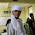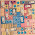Don't miss

## Problem From International Mathematical Olympiad (IMO) 2013

 Ditulis Oleh Unknown pada 27 July 2013 Tweet

Berikut soal International Mathematical Olympiad (IMO) ke- 54 tahun 2013 yang dilaksanakan di Colombia tanggal 23-24 Juli.

Day 1

Problem 1. Prove that for any two positive integers $k,n$ there exist positive integers $m_1,m_2,m_3,\cdots,m_k$ such that $$\begin{equation*} 1+\frac{2^k-1}{n}=\left(1+\frac{1}{m_1}\right)\left(1+\frac{1}{m_2}\right)\cdots\left(1+\frac{1}{m_k}\right) \end{equation*}$$

Problem 2. Given 2013 red and 2014 blue points in the plane, no three of them on a line. We aim to split the plane by lines (not passing through these points) into regions such that there are no regions containing points of both the colors. What is the least number of lines that always suffice?

Problem 3. Let $ABC$ be a triangle and let $A_1, B_1$, and $C_1$ be points of contact of the excircles with the sides $BC, AC$, and $AB$, respectively. Prove that if the circumcenter of $\triangle A_1B_1C_1$ lies on the circumcircle of $\triangle ABC$, then $\triangle ABC$ is a right triangle.

Day 2

Problem 4. Let $ABC$ be an acute triangle with orthocenter $H$, and let $W$ be a point on the side $BC$, between $B$ and $C$. The points $M$ and $N$ are the feet of the altitudes drawn from $B$ and $C$, respectively. $\omega_1$ is the circumcircle of triangle $BWN$ , and $X$ is a point such that $WX$ is a diameter of $\omega_1$. Similarly, $\omega_2$ is the circumcircle of triangle $CWM$ , and $Y$ is a point such that $WY$ is a diameter of $\omega_2$. Show that the points $X, Y$ , and $H$ are collinear

Problem 5. Let $\mathbb{Q}_{> 0}$ be the set of all rational numbers greater than zero. Let $f : \mathbb{Q}_{ > 0} \rightarrow \mathbb{R}$ be a function satisfying the following conditions :
(i). $f(x)f(y)\geq f(xy)$ for all $x,y\in \mathbb{Q}_{> 0}$
(ii). $f(x+y)\geq f(x)+f(y)$ for all $x,y\in \mathbb{Q}_{> 0}$
(iii). There exists a rational number $a > 1$ such that $f(a)=a$.
Show that $f(x)=x$ for all $x,y\in \mathbb{Q}_{> 0}$

Problem 6. Let $n\geq 3$ be an integer, and consider a circle with $n + 1$ equally spaced points marked on it. Consider all labellings of these points with the numbers $0, 1, \cdots, n$ such that each label is used exactly once; two such labellings are considered to be the same if one can be obtained from the other by a rotation of the circle. A labelling is called beautiful if, for any four labels $a < b < c < d$ with $a + d = b + c$, the chord joining the points labelled $a$ and $d$ does not intersect the chord joining the points labelled $b$ and $c$.
Let $M$ be the number of beautiful labellings and let $N$ be the number of ordered pairs $(x, y)$ of positive integers such that $x + y\leq n$ and $GCD(x, y) = 1$. Prove that $$\begin{equation*} M=N+1 \end{equation*}$$

1.1.